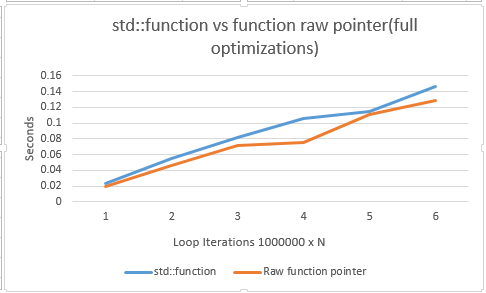# MechaTechnica

C++ 11 function pointersIntroduction
Function callbacks or function pointers in C/C++ were done by specifying the signature and name of the function. This led to ugly syntax. C++ 11 has simplified how function pointers are expressed by wrapping them into objects.

Function Pointers pre-C++ 11
Before C++ 11, we specified function pointers like the following
Example 1:
//function name and signature
typedef float (*twoArgFuncPtr)(float, float);
//a function matching the signature of the typedef function
float calledByPtr(float p1, float p2)
{
return (float)log(p2 / p1);
}
int main()
{
//fnc is a twoArgFuncPtr type which is
//assigned the calledByPtr function
twoArgFuncPtr fnc= calledByPtr;
std::cout<<fnc(2.3, 8.2);
return 0;
}
where the syntax:
ReturnType (*fncPtrName)(ArgType1, ArgType2...)
specified the function signature and its pointer name. We could then use fncPtrName as a type and assign to it any function that matches its signature.

Now consider member functions
Example 2:

float (MemberFnc::*myFuncPtr)(float,float);

class MemberFnc{
public:
float Db(float p1, float p2)
{
return (float)log(p2/p1);
}
};

int main()
{
MemberFnc memberFnc;
myFuncPtr= &MemberFnc::Db;
//this isn't pretty
std::cout<<((memberFnc).*(myFuncPtr))(2.1,2.3);
return 0;
}

The syntax for member function names was a little bit more involved:
ReturnType (ClassName::*fncPtrName)(ArgType1, ArgType2...);
However, calling it is even uglier:
((objectName).*(functionPtrName))(Arg1, Arg2..);

Enter C++ 11
In C++ 11, function pointers have been simplified a bit.
Example 3:
float (MemberFnc::*myFuncPtr)(float,float);
int main()
{
std::function<float(float,float)> newArgFuncPtr= calledByPtr;
std::cout<<newArgFuncPtr(2.3, 8.2);
return 0;
}
Now the new syntax is:
std::function<ReturnType(ArgType1, ArgType2) obj= functionName;

Example 4:
class MemberFnc{
public:
float Db(float p1, float p2)
{
return (float)log(p2/p1);
}
};

int main()
{
//will not compile in VS 2012 (bug)
std::function<float (MemberFnc&, float, float)> call_foo1 = &MemberFnc::Db;
return 0;
}

As mentioned in the comment, there is a bug in Visual Studio 2012. After searching online, it seems specific to 2012 so you may get this to work with other versions of VS. In GCC, it should work but I have not tested.

Apart from having a more readable syntax, what has been gained in using std::function as a wrapper?
• Ability to hold lambda expressions
• Wrap any callable function into one common object (i.e a vector of std::functions)
• Use of functors
• Use with std::bind
Performance
Is there a performance price to pay by using std::function? When full optimizations are turned on, there is not much performance cost to std::function (see the chart bellow).
Consider the following code:
#include<iostream>

typedef float (*twoArgFuncPtr)(float, float);

//function name and signature
typedef float (*twoArgFuncPtr)(float, float);
//a function matching the signature of the typedef function
float decibels(float p1, float p2)
{
return (float)log(p2 / p1);
}
int main()
{
typedef std::chrono::high_resolution_clock high_resolution_clock;
typedef std::chrono::milliseconds milliseconds;
twoArgFuncPtr fnc= decibels;
std::function<float(float,float)> fncObj= decibels;
const int numLoops= 1000000*2; //re-compiled for 1000000 * to N=6
high_resolution_clock::time_point start= high_resolution_clock::now();
float result=0;
for(int i=1, j=numLoops; i < numLoops-1; i++, j--)
{
//toggle the comment between the next two lines
//result+= fnc((float)i, (float)j); //call by raw ptr
result+= fncObj((float)i, (float)j);
}
high_resolution_clock::time_point end= high_resolution_clock::now();
std::cout<<"Time in ms: "<<
std::chrono::duration_cast<milliseconds>(end-start).count()<<std::endl;
std::cout<<"Result: "<<result<<std::endl;
return 0;
}

And the timings produced for a fully optimized program:At worst case scenario, std::function costs 10% more than raw pointers. You will have to re-compile the code when switching between the two function. I didn't automate the testing to avoid CPU caching of the inner loop.
Conclusion
I have not covered functors and lambda expressions in this post but I will definitely do it in the future. Given the low overhead of std::function and the having being more syntax friendly, I would recommend you use std::funciton from now on if you haven't already. Any thoughts on std::function? Write them in the comment below.

References
 http://c.learncodethehardway.org/book/ex18.html
 http://www.dreamincode.net/forums/topic/264061-c11-fun-with-functions/
 http://en.cppreference.com/w/cpp/utility/functional/function

Article Owner: RobertoOrellana

Article Creation Date: 7/12/2015 12:00:00 AM

Tags: No server implementation yet

Rating: 0